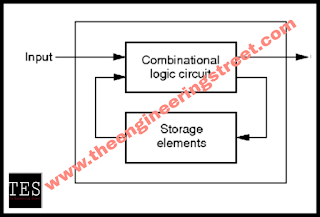# Sequential And Combinational Logic Circuits

## Sequential And Combinational Logic Circuits:

### The main part of digital circuits are sequential circuit and combinational logic circuit.

#### The sequential circuit:

Sequential circuits are circuits which is dependent on clock cycle and depends on present as well as past inputs to generates any output.sequential circuit

There are two types of sequential circuits i.e. asynchronous and synchronous circuits.

1)Synchronous: In synchronous circuits outputs change at specific time.

2)Asynchronous:outputs change at ant time.

A class of sequential circuits,they can be:

Bistable (2 stable states)
Monostable (1 stable state)
Astable  (no stable state)

Latches and flip flops are bistable logic devices. so latches and flip flops are sequential logic devices.

Main difference of them is method used for changing their state.

Two types of triggering or activation:Triggering means making a circuit active.

1) Level- triggering
•     latches
•     ON=1,OFF=0 ,(HIGH level,LOW level)
2) edge- triggering
•   flip flops
•   positive edge triggering(ON =from 0 to 1, OFF= other time)
•   negative edge triggering(ON= from 1 to 0, OFF= other time)

### Flip flops:

The flip-flop is the basic unit of digital memory. A flip-flop can remember one bit of data.

Sets of flip-flops are called registers, and can hold bytes of data.

By sending a signal to flip flops ,state can be changed.

There were number of  types of flip flops are SR flip flop ,D flip flop,T flip flop, JK flip flop.

1) SR flip flop:

Combinational logic circuits are define as a time independent circuits which do not depends on previous input to generates any outputs.combinational circuit

Combinational Circuits (CC) are circuits made up of different types of logic gates. A logic gate is a basic building block of any electronic circuit.

The output of the combinational circuit depends on the values at the input at any given time. The circuits do not make use of any memory or storage device.

There are three types of combinational circuits,shows as below.....

Arithmetic and logical combinational circuits – Adders , Subtractors, Multipliers, Comparators.

Data handling combinational circuits – Multiplexers, Demultiplexers, encoders, decoders.

Code converting combinational circuits – Binary to Gray, Gray to Binary, Binary to Excess 3,seven segment etc.

May You Like To Read This More Related Informative Articles On Electronics Engineering :